# Axis Customization in Blazor Stock Chart Component

17 Nov 202312 minutes to read

## Title

A title can be added to the axis using the Title property to provide quick information to the user about the data plotted in the axis. Title style can be customized using the TitleStyle property of the axis.

``````@using Syncfusion.Blazor.Charts

<SfStockChart Title="AAPL Stock Price">
<StockChartSeriesCollection>
<StockChartSeries DataSource="@StockDetails" Type="ChartSeriesType.Line" XName="Date" YName="Y"></StockChartSeries>
</StockChartSeriesCollection>
</SfStockChart>

@code {

public class ChartData
{
public DateTime Date { get; set; }
public Double Y { get; set; }
}

public List<ChartData> StockDetails = new List<ChartData>
{
new ChartData { Date = new DateTime(2012, 04, 02), Y= 100},
new ChartData { Date = new DateTime(2012, 04, 09), Y= 10},
new ChartData { Date = new DateTime(2012, 04, 16), Y= 500},
new ChartData { Date = new DateTime(2012, 04, 23), Y= 80},
new ChartData { Date = new DateTime(2012, 04, 30), Y= 200},
new ChartData { Date = new DateTime(2012, 05, 07), Y= 600},
new ChartData { Date = new DateTime(2012, 05, 14), Y= 50},
new ChartData { Date = new DateTime(2012, 05, 21), Y= 700},
new ChartData { Date = new DateTime(2012, 05, 28), Y= 90}
};
}``````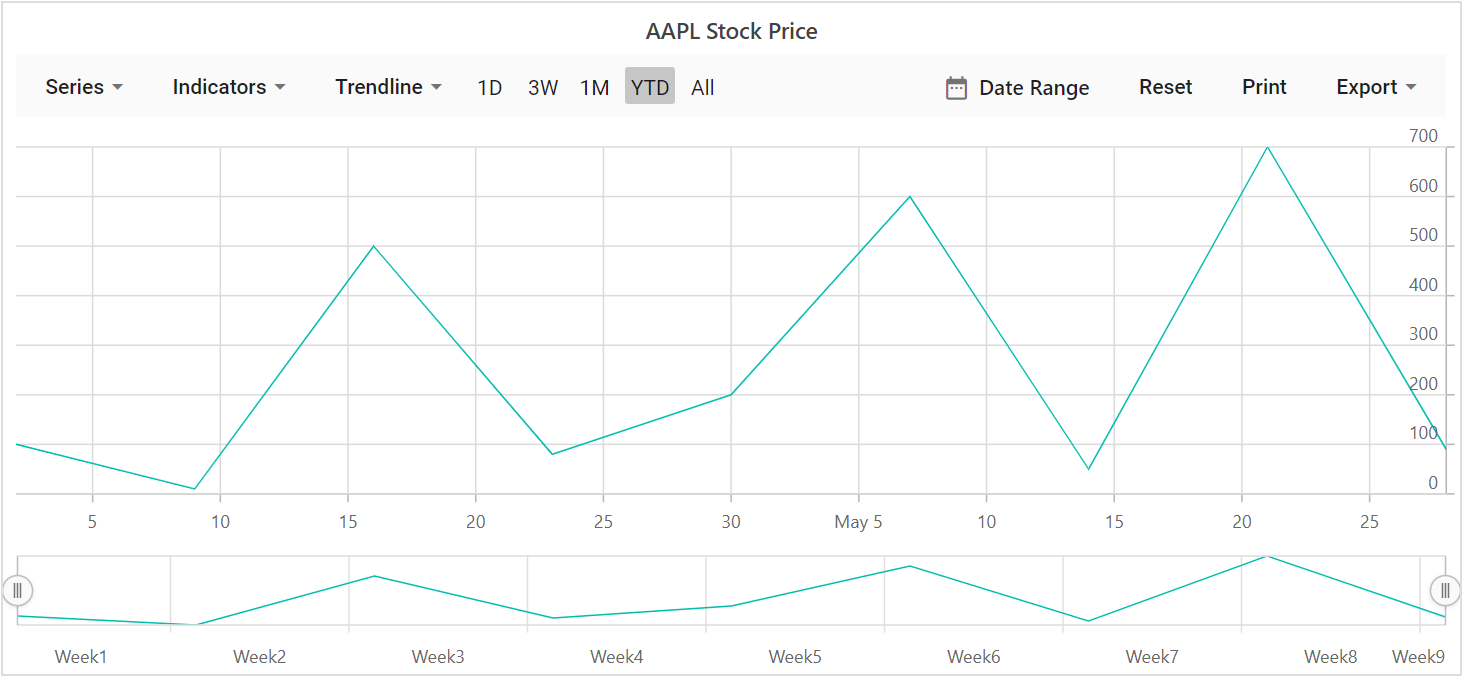## Tick Lines Customization

The `Width``Color` and `Size` of the minor and major tick lines can be customized using the MajorTickLines and the MinorTickLines properties in the axis.

``````@using Syncfusion.Blazor.Charts

<SfStockChart Title="AAPL Stock Price">
<StockChartPrimaryXAxis>
<StockChartAxisMajorTickLines Width="5" Color="blue"></StockChartAxisMajorTickLines>
<StockChartAxisMinorTickLines Width="0" Color="red"></StockChartAxisMinorTickLines>
</StockChartPrimaryXAxis>

<StockChartSeriesCollection>
<StockChartSeries DataSource="@StockDetails" Type="ChartSeriesType.Line" XName="Date" YName="Y"></StockChartSeries>
</StockChartSeriesCollection>
</SfStockChart>

@code {

public class ChartData
{
public DateTime Date { get; set;}
public Double Y { get; set;}
}

public List<ChartData> StockDetails = new List<ChartData>
{
new ChartData { Date = new DateTime(2012, 04, 02), Y= 100},
new ChartData { Date = new DateTime(2012, 04, 09), Y= 10},
new ChartData { Date = new DateTime(2012, 04, 16), Y= 500},
new ChartData { Date = new DateTime(2012, 04, 23), Y= 80},
new ChartData { Date = new DateTime(2012, 04, 30), Y= 200},
new ChartData { Date = new DateTime(2012, 05, 07), Y= 600},
new ChartData { Date = new DateTime(2012, 05, 14), Y= 50},
new ChartData { Date = new DateTime(2012, 05, 21), Y= 700},
new ChartData { Date = new DateTime(2012, 05, 28), Y= 90}
};
}``````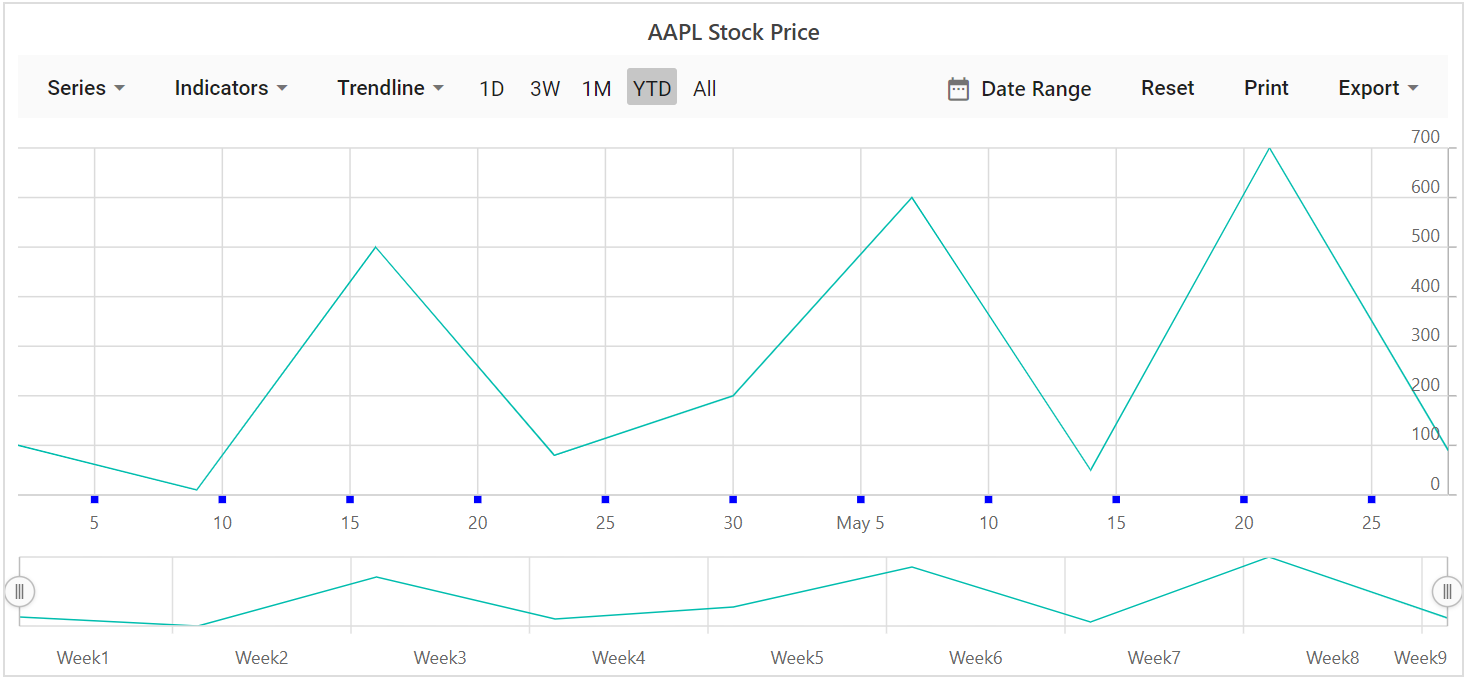## Grid Lines Customization

The `Width``Color` and `DashArray` of the minor and major grid lines can be customized using the MajorGridLines and the MinorGridLines properties in the axis.

``````@using Syncfusion.Blazor.Charts

<SfStockChart Title="AAPL Stock Price">
<StockChartPrimaryXAxis>
<StockChartAxisMajorGridLines Width="1" Color="blue"></StockChartAxisMajorGridLines>
<StockChartAxisMinorGridLines Width="0" Color="red"></StockChartAxisMinorGridLines>
</StockChartPrimaryXAxis>

<StockChartPrimaryYAxis>
<StockChartAxisMajorGridLines Width="1" Color="green"></StockChartAxisMajorGridLines>
<StockChartAxisMinorGridLines Width="0" Color="red"></StockChartAxisMinorGridLines>
</StockChartPrimaryYAxis>

<StockChartSeriesCollection>
<StockChartSeries DataSource="@StockDetails" Type="ChartSeriesType.Line" XName="Date" YName="Y"></StockChartSeries>
</StockChartSeriesCollection>
</SfStockChart>

@code {

public class ChartData
{
public DateTime Date { get; set; }
public Double Y { get; set; }
}

public List<ChartData> StockDetails = new List<ChartData>
{
new ChartData { Date = new DateTime(2012, 04, 02), Y= 100},
new ChartData { Date = new DateTime(2012, 04, 09), Y= 10},
new ChartData { Date = new DateTime(2012, 04, 16), Y= 500},
new ChartData { Date = new DateTime(2012, 04, 23), Y= 80},
new ChartData { Date = new DateTime(2012, 04, 30), Y= 200},
new ChartData { Date = new DateTime(2012, 05, 07), Y= 600},
new ChartData { Date = new DateTime(2012, 05, 14), Y= 50},
new ChartData { Date = new DateTime(2012, 05, 21), Y= 700},
new ChartData { Date = new DateTime(2012, 05, 28), Y= 90}
};
}``````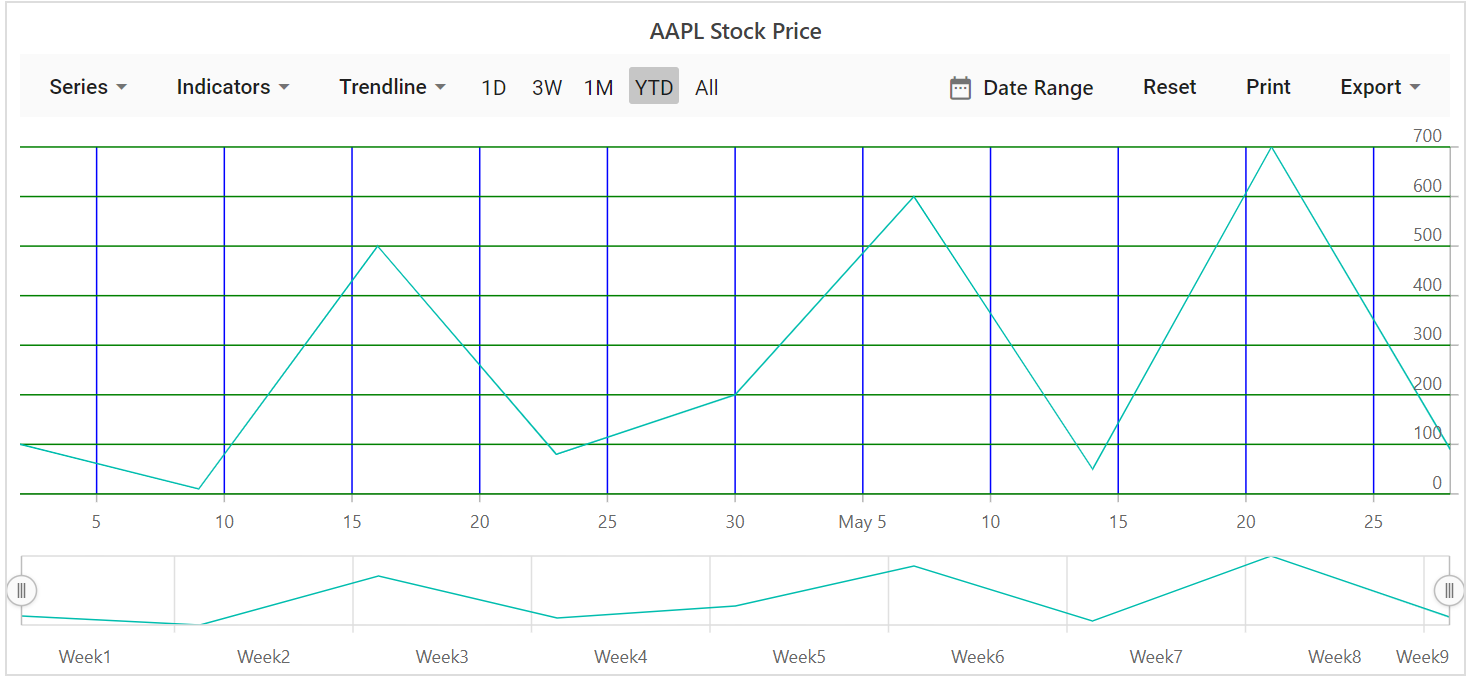## Multiple Axis

In addition to primary X and Y axis, n number of axis can be added to the chart. Series can be associated with this Axis, by mapping with axis’s unique name.

``````@using Syncfusion.Blazor.Charts

<SfStockChart Title="Multiple Axis">
<StockChartAxes>
<StockChartAxis RowIndex="0" Name="yAxis"></StockChartAxis>
</StockChartAxes>

<StockChartPrimaryXAxis>
<StockChartAxisMajorGridLines Width="0"></StockChartAxisMajorGridLines>
</StockChartPrimaryXAxis>

<StockChartPrimaryYAxis Interval="40">
<StockChartAxisLineStyle Color="Transparent"></StockChartAxisLineStyle>
<StockChartAxisMajorTickLines Width="0" Color="Transparent"></StockChartAxisMajorTickLines>
</StockChartPrimaryYAxis>

<StockChartCrosshairSettings Enable="true"></StockChartCrosshairSettings>

<StockChartSeriesCollection>
<StockChartSeries DataSource="@StockDetails" Type="ChartSeriesType.Candle" XName="Date" High="High" Low="Low" Open="Open" Close="Close" Name="Apple"></StockChartSeries>
<StockChartSeries DataSource="@StockDetails" Type="ChartSeriesType.Column" YAxisName="yAxis" XName="Date" YName="Low" Name="Google"></StockChartSeries>
</StockChartSeriesCollection>
</SfStockChart>

@code {

public class ChartData
{
public DateTime Date { get; set; }
public Double Open { get; set; }
public Double Low { get; set; }
public Double Close { get; set; }
public Double High { get; set; }
public Double Volume { get; set; }
}

public List<ChartData> StockDetails = new List<ChartData>
{
new ChartData { Date = new DateTime(2012, 04, 02), Open= 85.9757, High = 90.6657,Low = 85.7685, Close = 90.5257,Volume = 660187068},
new ChartData { Date = new DateTime(2012, 04, 09), Open= 89.4471, High = 92,Low = 86.2157, Close = 86.4614,Volume = 912634864},
new ChartData { Date = new DateTime(2012, 04, 16), Open= 87.1514, High = 88.6071,Low = 81.4885, Close = 81.8543,Volume = 1221746066},
new ChartData { Date = new DateTime(2012, 04, 23), Open= 81.5157, High = 88.2857,Low = 79.2857, Close = 86.1428,Volume = 965935749},
new ChartData { Date = new DateTime(2012, 04, 30), Open= 85.4, High =  85.4857,Low = 80.7385, Close = 80.75,Volume = 615249365},
new ChartData { Date = new DateTime(2012, 05, 07), Open= 80.2143, High = 82.2685,Low = 79.8185, Close = 80.9585,Volume = 541742692},
new ChartData { Date = new DateTime(2012, 05, 14), Open= 80.3671, High = 81.0728,Low = 74.5971, Close = 75.7685,Volume = 708126233},
new ChartData { Date = new DateTime(2012, 05, 21), Open= 76.3571, High = 82.3571,Low = 76.2928, Close = 80.3271,Volume = 682076215},
new ChartData { Date = new DateTime(2012, 05, 28), Open= 81.5571, High = 83.0714,Low = 80.0743, Close = 80.1414,Volume = 480059584}
};
}``````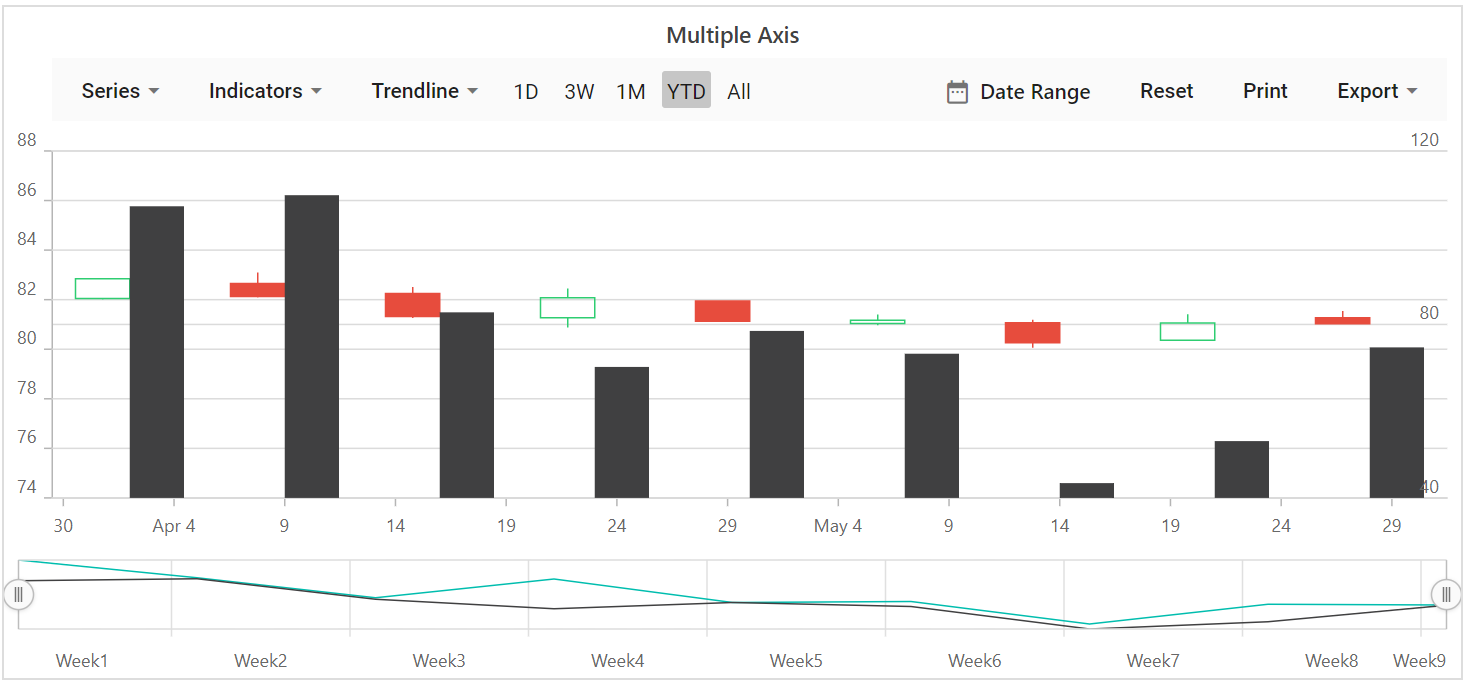## Inversed Axis

When an axis is inversed, highest value of the axis comes closer to origin and vice versa. To place an axis in inversed manner set this property IsInversed to `true`.

``````@using Syncfusion.Blazor.Charts

<SfStockChart Title="Inversed Axis">
<StockChartPrimaryXAxis IsInversed="true"></StockChartPrimaryXAxis>

<StockChartSeriesCollection>
<StockChartSeries DataSource="@StockDetails" Type="ChartSeriesType.Candle" XName="Date" High="High" Low="Low" Open="Open" Close="Close" Name="Apple"></StockChartSeries>
</StockChartSeriesCollection>
</SfStockChart>

@code {

public class ChartData
{
public DateTime Date { get; set; }
public Double Open { get; set; }
public Double Low { get; set; }
public Double Close { get; set; }
public Double High { get; set; }
public Double Volume { get; set; }
}

public List<ChartData> StockDetails = new List<ChartData>
{
new ChartData { Date = new DateTime(2012, 04, 02), Open= 85.9757, High = 90.6657,Low = 85.7685, Close = 90.5257,Volume = 660187068},
new ChartData { Date = new DateTime(2012, 04, 09), Open= 89.4471, High = 92,Low = 86.2157, Close = 86.4614,Volume = 912634864},
new ChartData { Date = new DateTime(2012, 04, 16), Open= 87.1514, High = 88.6071,Low = 81.4885, Close = 81.8543,Volume = 1221746066},
new ChartData { Date = new DateTime(2012, 04, 23), Open= 81.5157, High = 88.2857,Low = 79.2857, Close = 86.1428,Volume = 965935749},
new ChartData { Date = new DateTime(2012, 04, 30), Open= 85.4, High =  85.4857,Low = 80.7385, Close = 80.75,Volume = 615249365},
new ChartData { Date = new DateTime(2012, 05, 07), Open= 80.2143, High = 82.2685,Low = 79.8185, Close = 80.9585,Volume = 541742692},
new ChartData { Date = new DateTime(2012, 05, 14), Open= 80.3671, High = 81.0728,Low = 74.5971, Close = 75.7685,Volume = 708126233},
new ChartData { Date = new DateTime(2012, 05, 21), Open= 76.3571, High = 82.3571,Low = 76.2928, Close = 80.3271,Volume = 682076215},
new ChartData { Date = new DateTime(2012, 05, 28), Open= 81.5571, High = 83.0714,Low = 80.0743, Close = 80.1414,Volume = 480059584}
};
}``````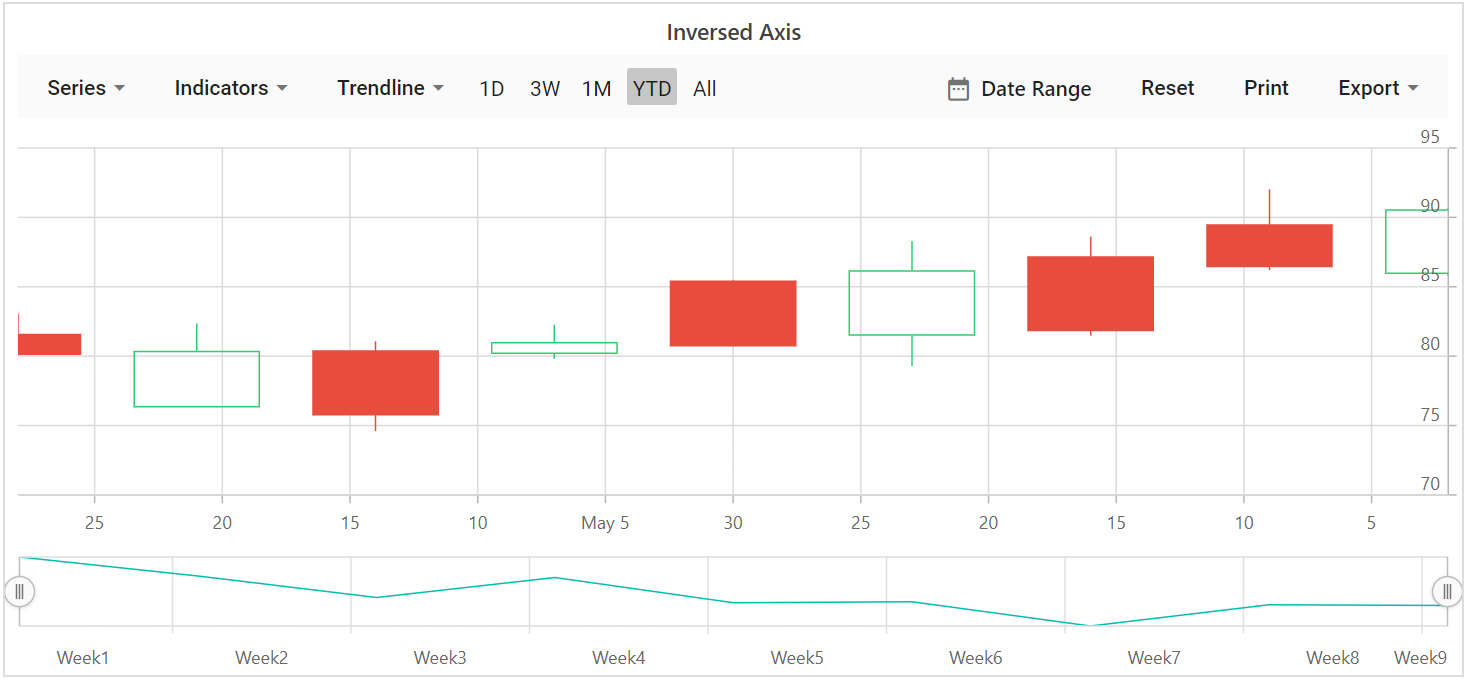## Opposed Position

To place an axis opposite from its original position, set OpposedPosition to true.

``````@using Syncfusion.Blazor.Charts

<SfStockChart Title="AAPL Stock Price">
<StockChartPrimaryXAxis OpposedPosition="true"></StockChartPrimaryXAxis>

<StockChartSeriesCollection>
<StockChartSeries DataSource="@StockDetails" Type="ChartSeriesType.Line" XName="Date" High="High" Low="Low" Open="Open" Close="Close"></StockChartSeries>
</StockChartSeriesCollection>
</SfStockChart>

@code {

public class ChartData
{
public DateTime Date { get; set; }
public Double Open { get; set; }
public Double Low { get; set; }
public Double Close { get; set; }
public Double High { get; set; }
public Double Volume { get; set; }
}

public List<ChartData> StockDetails = new List<ChartData>
{
new ChartData { Date = new DateTime(2012, 04, 02), Open= 85.9757, High = 90.6657,Low = 85.7685, Close = 90.5257,Volume = 660187068},
new ChartData { Date = new DateTime(2012, 04, 09), Open= 89.4471, High = 92,Low = 86.2157, Close = 86.4614,Volume = 912634864},
new ChartData { Date = new DateTime(2012, 04, 16), Open= 87.1514, High = 88.6071,Low = 81.4885, Close = 81.8543,Volume = 1221746066},
new ChartData { Date = new DateTime(2012, 04, 23), Open= 81.5157, High = 88.2857,Low = 79.2857, Close = 86.1428,Volume = 965935749},
new ChartData { Date = new DateTime(2012, 04, 30), Open= 85.4, High =  85.4857,Low = 80.7385, Close = 80.75,Volume = 615249365},
new ChartData { Date = new DateTime(2012, 05, 07), Open= 80.2143, High = 82.2685,Low = 79.8185, Close = 80.9585,Volume = 541742692},
new ChartData { Date = new DateTime(2012, 05, 14), Open= 80.3671, High = 81.0728,Low = 74.5971, Close = 75.7685,Volume = 708126233},
new ChartData { Date = new DateTime(2012, 05, 21), Open= 76.3571, High = 82.3571,Low = 76.2928, Close = 80.3271,Volume = 682076215},
new ChartData { Date = new DateTime(2012, 05, 28), Open= 81.5571, High = 83.0714,Low = 80.0743, Close = 80.1414,Volume = 480059584}
};
}``````